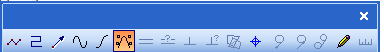# Control Curve

Draw / Lines / Control Curve

Toolbox Icon:Control Curve command draws a Nurb curve, whose shape is controlled by a 'cage' of control points. The curve can be selected to follow a quadratic or cubic rule; quadratic curves conform to the cage more closely than their cubic counterparts (i.e. cubic curves bend more 'stiffly' than quadratics).

As with the classic Curve command, a duplicate vertex in the cage polygon causes a sharp break in a quadratic control curve, or a much tighter bend in a cubic control curve.

This command is accessible via BasicCAD through the >ControlCurve command. You can specify whether the curve is Quadratic or Cubic via the <Cubic n parameter, where n=0 gives a quadratic result, and n=1 gives a cubic result.

Here is a simple example:

>ControlCurve

{

<Type 0 'normal, not vectorized

<Cubic 1 'cubic variation of curve

<PointXYZ 0, 0, 0

<PointXYZ 10, 0, 0

<PointXYZ 10, 10, 0

<Pointxyx 5, 15, 0

<Pointxyz 5, 15, 0 'sharp bend

<PointXYZ 0, 10, 0

<PointXYZ 0, 0, 0

}